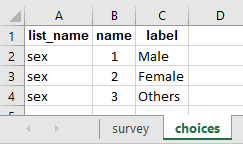# How do i calculate the values from within a repeat group?

Dear Sir,

Q1. Age
Q2. Sex (Male, Female, Others)

Q3. 0-18 Years Male (if the age filed is below and equal to 18 and Sex Field is equal to male, Then the value must be greater than 1)
Q4. 0-18 Years Female (if the age filed is below and equal to 18 and Sex Field is equal to Female, Then the value must be greater than 1)
Q5. 19 and above Male (if the age filed is equal to and above 19 and Sex Field is equal to male, Then the value must be greater than 1)
Q6. 19 and above Female (if the age filed is equal to and above 19 and Sex Field is equal to Female, Then the value must be greater than 1)

Hi @sarbeswar,

Is this setting under a repeat group (roster)? And do you wish to sum the total after you assign the values? Could you clarify these so that the solution could be provided in a single go.

Have a great day!

Hi,
I took the liberty of slightly amending your logic to make it fit a computation of the values from Q3 to Q6 instead of entering them. I also considered that you would make Q1 and Q2 repeated based on the number of people you would be asking that question (I am assuming a household). This is executed as below

The behavior, when deployed, is as follows

See the form here usertest08062020.xlsx (11.8 KB)

Stephane

1 Like

Thanks a lot,

But my question was

Q1. Age
Q2. Sex (Male, Female, Others)

Q3. 0-18 Years Male (User can enter the value but the condition would be, if the age filed is below and equal to 18 and Sex Field is equal to male, Then the value must be greater than 1)
Q4. 0-18 Years Female (User can enter the value but the condition would be if the age filed is below and equal to 18 and Sex Field is equal to Female, Then the value must be greater than 1)
Q5. 19 and above Male (User can enter the value but the condition would be if the age filed is equal to and above 19 and Sex Field is equal to male, Then the value must be greater than 1)
Q6. 19 and above Female (User can enter the value but the condition would be, if the age filed is equal to and above 19 and Sex Field is equal to Female, Then the value must be greater than 1)

User can enter the value in all the field but with this condition

Hi @sarbeswar,

If this is your requirement, you could simply delete the `read_only` columns (as shown in the image below). Doing so, you should be able to enter the fields.

Have a great day!

No sir I have already tried. But I want the Q3, Q4, Q5 and Q6 values must not be less then with the logic of Q1 and Q2

I mean to say that
While entering the value in the field (Q3, Q4, Q5, Q6) the value must not be smaller then with the entered value and logic given in Q1 and Qu.

For Example
If Q1 = 24
Q2 = Male
Then the Q5 value must be equal to 1 and grater than 1.

Hi @sarbeswar,

Could you kindly explain it again as i did not get it correctly:

With the condition provided above, you get a value of 1 for Q5 so how do you set it to greater than 1 (could you explain your condition) so that we could have a look if it’s possible.

Have a great day!

Sorry
For Example
If Q1 = 24
Q2 = Male
Then the Q5 value must be equal to 1 or greater than 1.

If Q1 = 18
Q2 = Female
Then the Q4 value must be equal to 1 or greater than 1

Hi @sarbeswar,

Could you share with us a screenshot (Enketo) of what you assume wrong while entering a data . Maybe we understand your issue through he screenshot.

Have a great day!

Dear Sir

I am conducting a household survey. The format is
Q1 = Name of HH
Q2 = Age of HH
Q3 = Sex (Male/ Female)

Family Member Details
Q4 = How many Boys (0 - 18)
Q5 = How many Girls (0 - 18)
Q6 = How many Male (19 & Above)
Q7 = How many Female (19 & Above)

If the HH age is 45 and Male. In his family another 5 members also there.
The details are - Boys (0 - 18) is 1
Girls (0 - 18) is 1
Male (19 & Above) is 2
Female (19 & Above) is 1

Then I want to validate the data with all the columns with age and sex of HH.
Here if someone is entering the data then the Q6 field must be greater than 1. Because the Head of the HH is 45 and male. So automatically Q6 column will come with 1 and if want then the number may be increased but never be zero (0).

As I understood from your post, you are looking for constraints not calculation.
The constraints, based on your criteria should be like this:

Please find attached file: usertest08062020.xlsx (11.5 KB)
Best,

Yasser

2 Likes

Thank you so much.

Actually this is what I was looking for.

2 Likes

Hi @sarbeswar,

Backing up with what @stephanealoo has outlined (i.e. calculating within a repeat group) and also what @Ysr3322 has outlined (i.e. using constraint to a grouping question), the same can be done for a repeat group with a constraint. Detailing it out so that some users could be benefited from the same.

### In the choices tab of your xlsform:### Reference xlsform:

usertest08062020_ed.xlsx (12.5 KB)

Have a great day!

2 Likes